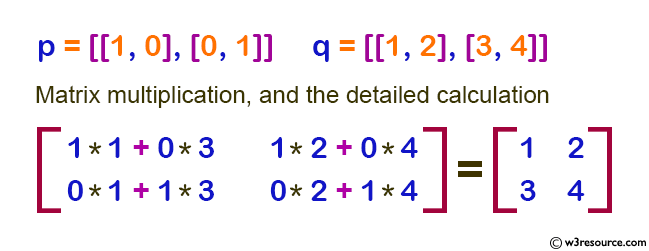﻿ NumPy: Compute the multiplication of two given matrixes - w3resource# NumPy: Compute the multiplication of two given matrixes

## NumPy: Linear Algebra Exercise-1 with Solution

Write a NumPy program to compute the multiplication of two given matrixes.

Sample Matrix:

```[[1, 0], [0, 1]]
[[1, 2], [3, 4]]```

Matrix multiplication, and the detailed calculation:Sample Solution :

Python Code :

``````import numpy as np
p = [[1, 0], [0, 1]]
q = [[1, 2], [3, 4]]
print("original matrix:")
print(p)
print(q)
result1 = np.dot(p, q)
print("Result of the said matrix multiplication:")
print(result1)
print(result2)
``````

Sample Output:

```original matrix:
[[1, 0], [0, 1]]
[[1, 2], [3, 4]]
Result of the said matrix multiplication:
[[1 2]
[3 4]]
```

Python Code Editor:

Have another way to solve this solution? Contribute your code (and comments) through Disqus.

What is the difficulty level of this exercise?

Test your Python skills with w3resource's quiz

﻿

## Python: Tips of the Day

```print(2_000_000)
```2000000